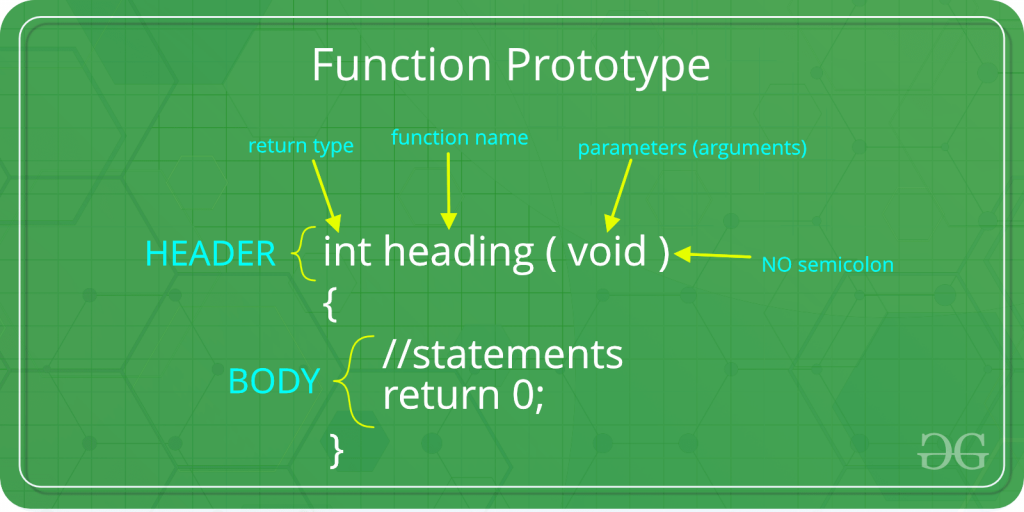# Argument Coercion in C/C++

Argument coercion is a feature of function prototypes by which the compiler implicitly converts the datatype of the arguments passed during the function call to match the datatype in the definition of the function.It follows Argument promotion rules. Therefore a lower datatype maybe converted into a higher datatype but vice-versa is not true. This is because when a higher datatype is converted to a lower datatype it can result into loss or truncation of data.

The Promotion hierarchy for fundamental datatypes in C++ is:Example: Take for Example the code given below consists of an add function that expects double arguments. But even when integer arguments are passed it works correctly. In case long double arguments are passed the code will give an error.

 `#include ` `using` `namespace` `std; ` ` `  `double` `add(``double` `a, ``double` `b) ` `{ ` `    ``return` `a + b; ` `} ` ` `  `int` `main() ` `{ ` `    ``// Passing double arguments, as expected ` `    ``cout << ``"Sum = "` `<< add(2.4, 8.5) << endl; ` ` `  `    ``// Passing int arguments, when double is expected ` `    ``// This will lead to Argument Coercion ` `    ``cout << ``"Sum = "` `<< add(16, 18) << endl; ` ` `  `    ``return` `0; ` `} `

Whether you're preparing for your first job interview or aiming to upskill in this ever-evolving tech landscape, GeeksforGeeks Courses are your key to success. We provide top-quality content at affordable prices, all geared towards accelerating your growth in a time-bound manner. Join the millions we've already empowered, and we're here to do the same for you. Don't miss out - check it out now!

Previous
Next